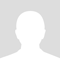# Direct Proportion and Inverse Proportion: Ball Throwing Experiment

In math or science we come across terms such as inverse proportion and direct proportion. When two variables are directly proportional an increase in one variable causes an increase in the other variable. When two variables are inversely proportional an increase in one variable causes a decrease in the other variable.

Inverse Proportion:
To illustrate inverse proportionality, I will use a common physics problem. Two golf balls are thrown down from a tall building at the same time and one ball has twice the velocity of the other ball. Which ball hits the ground first assuming only velocity is different?

We already know that velocity is approximately equal to distance / time.
Let the velocity of the slower ball be v. Assuming only the velocity of the two balls is different, we can say approximately v = d / t. We can eliminate wind force, atmospheric force, and force of gravity since both balls will be affected equally.
If you increase v, you are essentially reducing t, because they are inversely proportional.
So the faster ball(ball with twice the velocity) will hit the ground first. It will hit the ground in approximately half the time the slower ball takes.
So the consequences of inverse proportion is that if one variable in the relationship rises, the other variable plummets.

Direct Proportion:
Distance and velocity are directly proportional. So let's now assume that we measure the distance travelled by both balls 1 second later assuming the balls did not hit the ground yet. The faster ball will cover a longer distance first. This means keeping time constant, a rise in velocity will result in a corresponding rise in distance. This is because the two variables velocity and distance are directly proportional to each other.